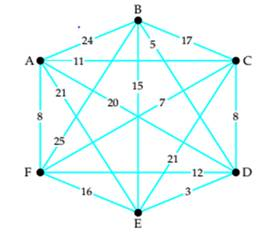# Use the greedy algorithm and the weighted graph below to generate a Hamiltonian circuit starting from each vertex. Then compare the different circuits to find the one of smallest total weight.### Mathematical Excursions (MindTap C...

4th Edition
Richard N. Aufmann + 3 others
Publisher: Cengage Learning
ISBN: 9781305965584

#### Solutions

Chapter
Section### Mathematical Excursions (MindTap C...

4th Edition
Richard N. Aufmann + 3 others
Publisher: Cengage Learning
ISBN: 9781305965584
Chapter 5.2, Problem 2EE
Textbook Problem
493 views

## Use the greedy algorithm and the weighted graph below to generate a Hamiltonian circuit starting from each vertex. Then compare the different circuits to find the one of smallest total weight.To determine

The Hamiltonian circuits using greedy algorithm starting at each vertex. Then compare the various Hamiltonian circuits to identify the one with the smallest total weight.

### Explanation of Solution

Given information:

The graph of Hamiltonian circuit is

Calculation:

1. Using Greedy algorithm, the Hamiltonian circuit starting at vertices A is:
2. The circuit starting at A: A − F − C − D − E − B − A.

Total weight of this circuit is 8 + 7 + 8 + 3 + 15 + 24 = 65

3. The Hamiltonian circuit starting at vertices B is:
4. The circuit starting at B: B − D − E − F − C − A − B.

Total weight of this circuit is 5 + 3 + 16 + 7 + 11 + 24 = 66

5. The Hamiltonian circuit starting at vertices C is:
6. The circuit starting at C: C − F − A − D − E − B − C.

Total weight of this circuit is 7 + 8 + 20 + 3 + 15 + 17 = 70

7. The Hamiltonian circuit starting at vertices D is:
8. The circuit starting at D: D − E − B − C − F − A − D...

### Still sussing out bartleby?

Check out a sample textbook solution.

See a sample solution

#### The Solution to Your Study Problems

Bartleby provides explanations to thousands of textbook problems written by our experts, many with advanced degrees!

Get Started

Find more solutions based on key concepts
Identify the quadratic polynomial from among (a)-(c) and evaluate it using.

Mathematical Applications for the Management, Life, and Social Sciences

In Exercises 1-6, simplify the expression. 818

Calculus: An Applied Approach (MindTap Course List)

Explain in your own words the meaning of limxf(x)=L and limxf(x)=M.

Applied Calculus for the Managerial, Life, and Social Sciences: A Brief Approach

Let A= Show that (AT)1=(A1)T.

Finite Mathematics for the Managerial, Life, and Social Sciences

Find a definite integral for the volume of a solid with a circular base of radius 4 inches such that the cross ...

Study Guide for Stewart's Single Variable Calculus: Early Transcendentals, 8th

In Problems 120 determine whether the given differential equation is exact. If it is exact, solve it. 17. (tan ...

A First Course in Differential Equations with Modeling Applications (MindTap Course List)

Slope from the Rise and Run One end of a ladder is on the ground. The top of the ladder rests at the top of an ...

Functions and Change: A Modeling Approach to College Algebra (MindTap Course List)

Health insurance benefits vary by the size of the company (the Henry J. Kaiser Family Foundation website, June ...

Modern Business Statistics with Microsoft Office Excel (with XLSTAT Education Edition Printed Access Card) (MindTap Course List)